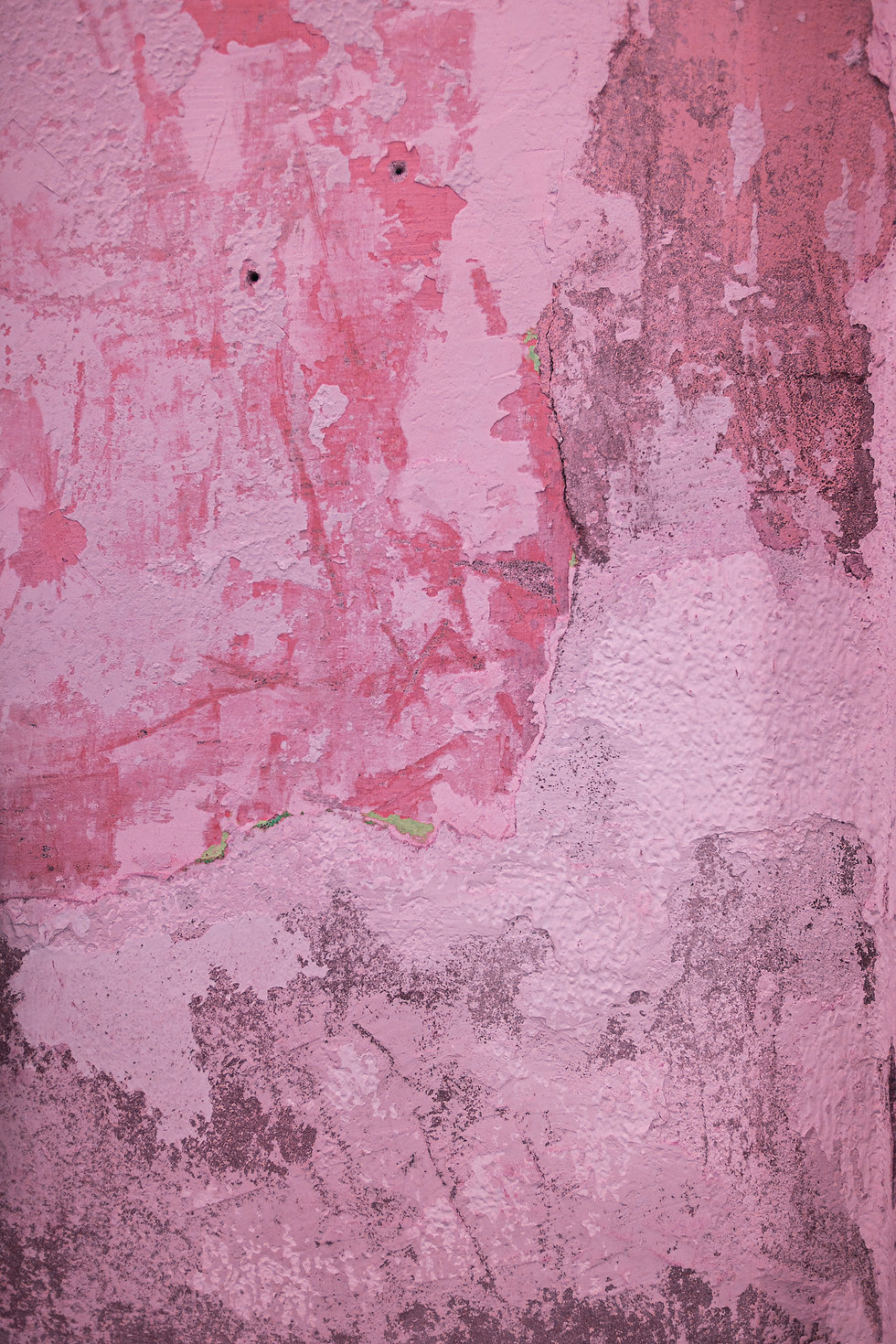VIEW IN FULL SCREEN

# AQA A-level Physics Stationary wavesSuperposition is the process by which two or more waves combine into a single waveform when they overlap. The total displacement at any point is equal to the vector sum of the individual displacements at that point on the waves that are overlapping. A standing or stationary wave is formed when two progressive waves which are travelling in opposite directions superpose to form a wave with constant points of zero amplitude called nodes where destructive interference occurs and the two waves meet in antiphase and points of maximum amplitude called antinodes where constructive interference occurs and the waves meet in phase. For a stationary wave to be formed by waves meeting, those two waves must have the following properties: same frequency, wavelength and amplitude, must be travelling in opposite directions. These conditions can also be met when a wave is reflected back onto itself. Energy is stored rather than transferred along a stationary wave.A stationary wave is made up of a repeating pattern of nodes and antinodes. Along a stationary wave, there are points that appear to be going through zero displacement. These points are called nodes, and is where the interfering waves are in antiphase (phase difference of π), meaning that destructive interference occurs. On the other hand, there are ponits along a stationary wave that undergo maximum displacement. These points are called antinodes, and is where the interfering waves are in phase (phase difference of 0), meaning that constructive interference occurs. The wavelength of a stationary wave can be found by doubling the distance between two consecutive nodes or two consecutive antinodes.When a string is plucked, a stationary wave is formed along it. The string can only vibrate at certain frequencies, which are called harmonics. The lowest possible frequency is when the string vibrates with just one loop. This frequency is called the fundamental frequency or the first harmonic. The wavelength of the wave is double the length of the string, ie. double the length between two nodes. For the second harmonic, another loop is added, halving the wavelength, which is now equal to the length of the string, and doubling the frequency. For the third harmonic, there are three loops, so the wavelength is a third of the one at fundamental frequency and the frequency is three times greater than it. This pattern continues for however many loops are added. The fundamental frequency of a string can be found using: f₁ = (1/2L) × √(T/μ), where L = length of string, T = tension in string and μ = mass per unit length.Stationary waves can form anywhere where two waves moving in opposite direction with equal wavelength, frequency and amplitude interfere. A notable example of this is in string and wind instruments. In string instruments, stationary waves are formed due to the plucking of the strings. When a wind instrument is blown into, a sound wave travels inside its pipe and is reflected at each end of the air column. The two waves superpose to create a stationary wave. Vibrating air columns can be either closed (closed at one end and open at the other) or open (open at both ends). A node is always formed at a closed end, since the air cannot freely vibrate, and an anti-node is formed at an open end, since the air can vibrate with maximum amplitude.# ✅

Draw a stationary wave and label one antinode and one node.# ✅

Explain why when a stationary wave is formed, nodes and antinodes are produced.# ✅

What must be the phase difference at a point where two waves are interfering for a node to be formed?# ✅

Given that the distance between two consecutive antinodes is 1.5 m, find the wavelength of the stationary wave.# ✅

State the points at which destructive interference occurs along the given stationary wave.# ✅

Given that the fundamental frequency of a string is 300 Hz, find the third harmonic.# ✅

The distance between two consecutive nodes on a string at its third harmonic is 0.4 m. Calculate the wavelength of the first harmonic.# ✅

The given string has a length of L and a fundamental frequency of f₁. State which harmonic is given in the diagram and find its wavelength and frequency at this harmonic.# ✅

Air is blown into an open pipe of length 1.2 m. Given that the wave oscillates at fundamental frequency, find its wavelength.# ✅

A sound wave is oscillating inside a closed pipe of length 2.2 m at its third harmonic. Find the wavelength of the sound wave.Have you found the questions useful?